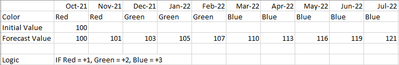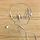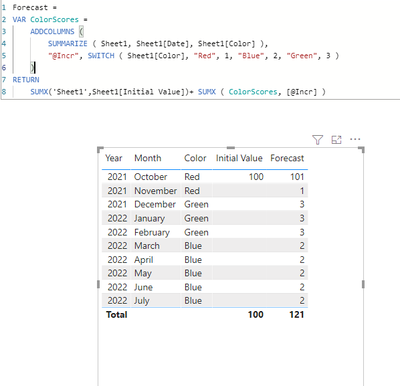cancel
Showing results for
Did you mean:

Fabric is Generally Available. Browse Fabric Presentations. Work towards your Fabric certification with the Cloud Skills Challenge.Frequent Visitor

## DAX calculation with incremental value

Hello,
I am trying to calculate the "Forecast Value" based on the "Color" below.
What would be the DAX formula for this?1 ACCEPTED SOLUTIONCommunity Support

I doubt you can do the logic and cumulative sum in one dax. You can create a logic column then get the forecast value in a measure.

forecastlogic column=
var logic = SWITCH([Color],"Red",1,"Green",2,"Blue",3)
Return IF([Initial Value]=100,100,logic)

Forcast value = CALCULATE(SUM('Table'[forecastlogic]),FILTER(ALL('Table'),[YearMonth]<=MAX([YearMonth])))Paul Zheng _ Community Support Team
If this post helps, please Accept it as the solution to help the other members find it more quickly.

7 REPLIES 7Community Support

I doubt you can do the logic and cumulative sum in one dax. You can create a logic column then get the forecast value in a measure.

forecastlogic column=
var logic = SWITCH([Color],"Red",1,"Green",2,"Blue",3)
Return IF([Initial Value]=100,100,logic)

Forcast value = CALCULATE(SUM('Table'[forecastlogic]),FILTER(ALL('Table'),[YearMonth]<=MAX([YearMonth])))Paul Zheng _ Community Support Team
If this post helps, please Accept it as the solution to help the other members find it more quickly.Super User

A calculated column is definitely recommended here but you can do without if you need to (e.g. if the color values are dynamic).

``````Forcast (AO) =
VAR MaxDate = MAX ( Table1[YearMonth] )
VAR ColorScores =
SUMMARIZE (
FILTER ( ALL ( Table1 ), Table1[YearMonth] <= MaxDate ),
Table1[YearMonth],
Table1[Color]
),
"@Init", CALCULATE ( SUM ( Table1[Initial Value] ) ),
"@Incr", SWITCH ( Table1[Color], "Red", 1, "Green", 2, "Blue", 3 )
)
RETURN
SUMX ( ColorScores, MAX ( [@Init], [@Incr] ) )``````

See attached.Super User

If you have one color per month, then you can calculate the final forecasted value over a selected period like this:

``````Forcast =
VAR ColorScores =
SUMMARIZE ( Table1, Table1[Month], Table1[Color] ),
"@Incr", SWITCH ( Table1[Color], "Red", 1, "Blue", 2, "Green", 3 )
)
RETURN
[InitialValue] + SUMX ( ColorScores, [@Incr] )``````Frequent Visitor

I don't think the above code works...
Am I missing something?Super User

The forecast total works as I intended. You have to do a bit more work to get the months to work like a cumulative total.

Something more like this:

``````Forcast =
VAR MaxDate = MAX ( Table1[Date] )
VAR InitialValue = CALCULATE ( SUM ( Table1[InitialValue] ), Table1[Date] <= MaxDate )
VAR ColorScores =
SUMMARIZE (
FILTER ( Table1, Table1[Date] <= MaxDate ),
Table1[Month],
Table1[Color]
),
"@Incr", SWITCH ( Table1[Color], "Red", 1, "Blue", 2, "Green", 3 )
)
RETURN
InitialValue + SUMX ( ColorScores, [@Incr] )``````Frequent Visitor

I am getting the same resultSuper User

Probably because you have Year and Month context in your visual rather than just Date.

You can wipe out all of the filter context with ALL.

``````Forcast =
VAR MaxDate =  MAX ( Table1[Date] )
VAR InitialValue =
CALCULATE (
SUM ( Table1[InitialValue] ),
FILTER ( ALL ( Table1 ), Table1[Date] <= MaxDate )
)
VAR ColorScores =
SUMMARIZE (
FILTER ( ALL ( Table1 ), Table1[Date] <= MaxDate ),
Table1[Month],
Table1[Color]
),
"@Incr", SWITCH ( Table1[Color], "Red", 1, "Blue", 2, "Green", 3 )
)
RETURN
InitialValue + SUMX ( ColorScores, [@Incr] )
``````Announcements#### Power BI Monthly Update - November 2023

Check out the November 2023 Power BI update to learn about new features.#### Fabric Community News unified experience

Read the latest Fabric Community announcements, including updates on Power BI, Synapse, Data Factory and Data Activator.#### Exclusive opportunity for Women!

Join us for a free, hands-on Microsoft workshop led by women trainers for women where you will learn how to build a Dashboard in a Day!#### The largest Power BI and Fabric virtual conference

130+ sessions, 130+ speakers, Product managers, MVPs, and experts. All about Power BI and Fabric. Attend online or watch the recordings.Top Solution Authors
Top Kudoed Authors
Users online (3,729)